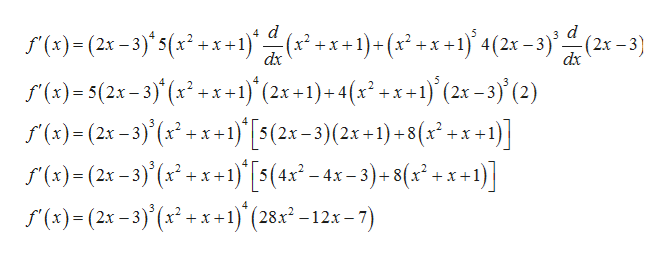# Find the derivative of the function.f(x) = (2x − 3)4(x2 + x + 1)5

Question
1 views

Find the derivative of the function.

f(x) = (2x − 3)4(x2 + x + 1)5
check_circle

Step 1

Given function is

Step 2

We will find f'(x) by using product rule of differentiation  , which states that if f=uv, then f'=uv'+vu'

Step 3

Now, we will use the result that derivative o...help_outlineImage Transcriptioncloser)-(2x-3's(x) x4(2-3) d (2-3) f ()-5(2x-3) (x2 + x+1) (2x +1)+ 4(x2 +x+1)} (2x -3)' (2) r(x)-(2x-3)(x)[5(2x -3)(2x -1)-8(x +x +1)] ()(2x-3) (x)'[5(4x -4x -3)-8( + x +1)] r(x)(2x-3) 1) (28 -12x -7) +x +1)+(x +x+14(2x -3)(2x -3) +4(x2 +x+1)(2x - 3) (2) = = _ fullscreen

### Want to see the full answer?

See Solution

#### Want to see this answer and more?

Solutions are written by subject experts who are available 24/7. Questions are typically answered within 1 hour.*

See Solution
*Response times may vary by subject and question.
Tagged in

### Calculus